2. Theory
2.1 Contour Dynamics
2.2 Equations of Cotour Dynamics
2.3 Theory of an Elliptical Vortex
2.4 Finite-Time Lyapunov Analysis on Fluid Deformation

### 2.4 Finite-Time Lyapunov Analysis on Fluid Deformation

Yoden and Nomura(1993) did a finite-time Lyapunov analysis in phase space. The same analysis is done here in physical space to introduce a linear measure of deformation of a fluid percel.

Trajectory of a fluid particle is given as a solution of the equation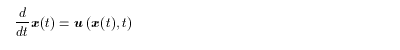(2.16)
Time evolution of a small perturbation from the solution can be described by a tangent linear equation of (2.16):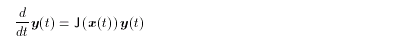(2.17)
where J is Jacobian matrix of u at x( t ).

By integrating (2.17) from t0 to t0 +t,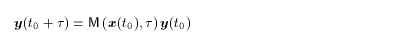(2.18)
where the matrix M is the resolvent.

Now, let us consider a small perturbation on the circle with a radius e around x( t0 ):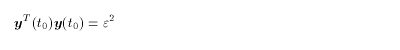(2.19)
Using (2.18) yields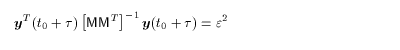(2.20)
which means that the circle is deformed into an ellipse (Fig. 1). The length and direction of the semi-axes of the ellipse are to be eigenvalues and eigenvectors of M MT, which is a semi-positive symmetric matrix.

Assuming that the eigenvalues of M MT are e2li( x(t0), t) and that the eigenvectors are fi ( x(t0), t), we obtain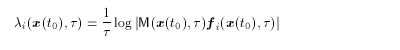(2.21)
which, combined with (2.18), shows that li( x(t0), τ) are the finite-time Lyapunov exponents and fi ( x(t0),t) the vectors. They become ordinary Lyapunov exponents and vectors if the limitation t®¥ is taken.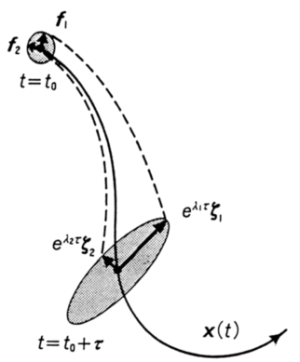Figure 1: Finite-time lyapunov analysis on fluid deformation.
The circle is deformed into the ellipse after a finite time t. The length of the semi-axes is given as elit.

<<index <previous next>